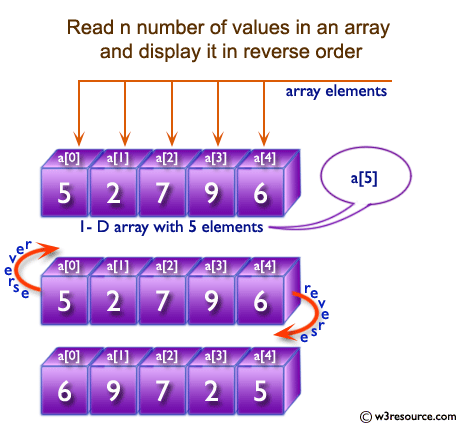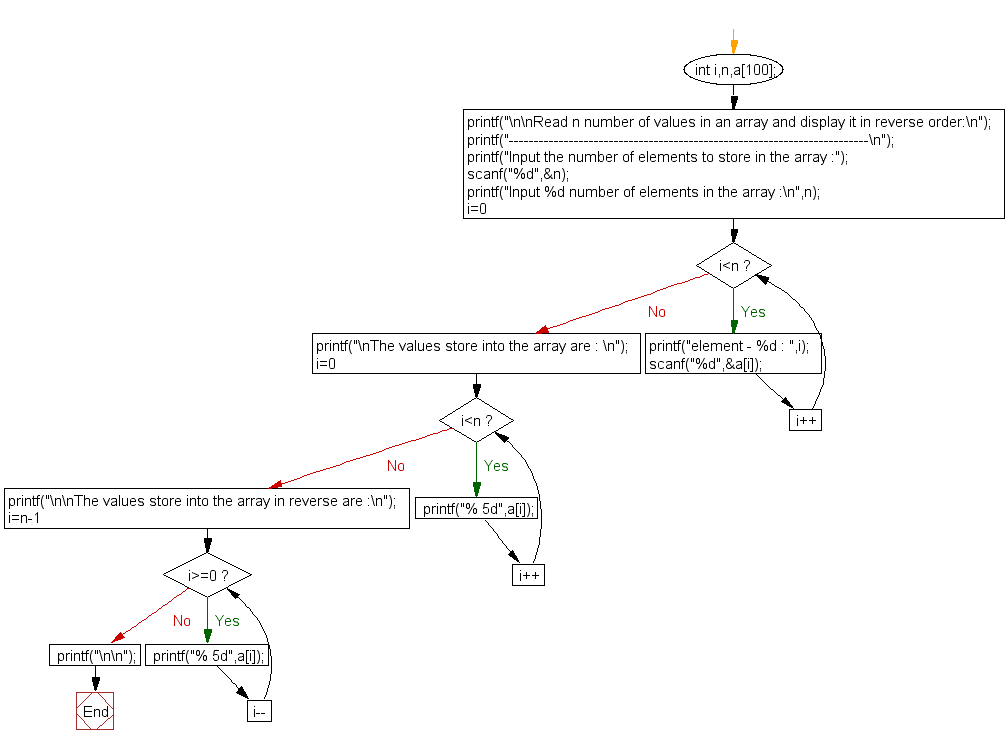﻿ C exercises: Read n number of values in an array and display it in reverse order - w3resource# C Exercises: Read n number of values in an array and display it in reverse order

## C Array: Exercise-2 with Solution

Write a program in C to read n number of values in an array and display it in reverse order.

Pictorial Presentation:Sample Solution:

C Code:

``````#include <stdio.h>

void main()
{
int i,n,a;

printf("\n\nRead n number of values in an array and display it in reverse order:\n");
printf("------------------------------------------------------------------------\n");

printf("Input the number of elements to store in the array :");
scanf("%d",&n);

printf("Input %d number of elements in the array :\n",n);
for(i=0;i<n;i++)
{
printf("element - %d : ",i);
scanf("%d",&a[i]);
}

printf("\nThe values store into the array are : \n");
for(i=0;i<n;i++)
{
printf("% 5d",a[i]);
}

printf("\n\nThe values store into the array in reverse are :\n");
for(i=n-1;i>=0;i--)
{
printf("% 5d",a[i]);
}
printf("\n\n");
}
```
```

Sample Output:

```Read n number of values in an array and display it in reverse order:
------------------------------------------------------------------------
Input the number of elements to store in the array :3
Input 3 number of elements in the array :
element - 0 : 2
element - 1 : 5
element - 2 : 7

The values store into the array are :
2    5    7

The values store into the array in reverse are :
7    5    2
```

Flowchart:C Programming Code Editor:

Improve this sample solution and post your code through Disqus.

What is the difficulty level of this exercise?

﻿